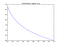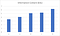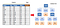# What is Data Mining?

Christian Zuniga, PhD

Sunday, February 14 2021

Data mining is what it says, mining data. Although this frequently involves accessing data from databases, this is only one step. Data mining ultimately seeks to extract non-obvious patterns from data of potential value. In other words, data mining extracts information from data. The amount of data being generated and recorded has exploded in the past decades. Decreasing cost of digital storage and transmission allows gathering more and more data in multiple forms such as images, video, and text. However, the amount of useful information may be much less. Finding information in data can be seen as finding diamonds in a sea of carbon. How can the value of data be assessed? One way is by using information theory.

What is information? It is what you don’t already know. If a piece of data doesn’t reveal anything new, or is certain, it contains no information . This measure of information was made by Claude Shannon in 1948 in his paper “A Mathematical Theory of Communication”. To measure information, data is considered as part of a larger set, but not a universal set of everything. For example, English letters form part of a set of 26 symbols (considering only lower case). Some symbols, like the letter ‘e’, occur more frequently than others. This probability of occurrence indicates how much information a symbol, or piece of data, has. If the symbol always happened, it has a probability of 1 and contains 0 information. This may seem a little counter-intuitive but makes sense after some thought. If you’re reading an article and know what letter comes next, you’re not obtaining new information. There is an inverse relationship between the probability, and the amount of information, or uncertainty, as shown in Figure 1. The lower the probability of a data item, the greater the amount of information, which has units of bits.Figure 1 Information in a data item obtained from the probability by log(1/p). A data item that is infrequent has probability close to 0, is more uncertain, and has more information if it occurred. The amount of information is measured in bits. If the data item has a probability of 0.1, it has about 3.3 bits of information.

Figure 2 shows the information content of the first five letters a, b, c, d, e. The letter ‘e’, being the most common, has the least amount of information. The average information of all the letters gives the entropy of the alphabet which has been estimated to be ~1.8 bits/letter .Figure 2 The information content of a, b , c, d, e calculated using the probability of each letter with respect to a larger alphabet. Letter ‘b’, being the least likely has about 6 bits of information.

Entropy is Shannon’s measure of information and represented by the letter H. The entropy gives the average amount of information in a collection of N data items. An item n has probability pn of occurring.

A bit is also used to refer to a binary digit, 1 or 0. However, bits in this context refers to amount of information, specifically it is related to the number of yes/no questions needed to determine what data item occurred. One binary digit may carry one bit of information or less. The size of a data item like a file or image, only loosely gives the amount of information in it.

Data mining then can be thought of as extracting bits of information. In prediction for example, the objective is to classify a data item into a category or predict a continuous value using the item’s features. If Y is the item’s class and X is a feature of the item, the information gain, H(Y|X), gives how many bits of information X gives about Y. It is equal to the original entropy H(Y) minus the mutual information between X and Y. The mutual information I(Y,X) is a straightforward generalization of the entropy formula to two variables.

A simple problem is given by the task of deciding to play golf on a given day, further explained in the reference . Figure 3 shows this set of data has 1 target variable, to play yes or no, and 4 features or predictors. This is a training set in which the target is known. The task is to use this set to make a model that predicts if given only the 4 features, whether to play golf or not. If you’re not a fan of golf, perhaps your spouse is. A decision tree is a simple, intuitive model that can give such a prediction. As shown the tree organizes the features to decide yes or no. Using the information gain gives a useful way to train the tree. The training process generally proceeds as follows.

1. Calculate the initial target entropy H(Play Golf) = 0.94 bits

2. Select predictor that gives the greatest information gain as the first deciding factor.

a. Outlook: Gives 0.247 bits of information and ‘Outlook’ feature is selected.

b. Temperature: gives 0.029 bits

c. Humidity: gives 0.152 bits

d. Windy: gives 0.048 bits

3. Consider only the remaining predictors and repeat step 2 for each of the values of the selected predictor.Figure 3 Decision tree used to decide to play golf or not. The most important feature used to decide to play is the Outlook, whether it is rainy, sunny, or overcast.

Although this is a simple example, it shows well the overall process of using information theory in classification. Information in bits, is extracted from the features to decide an outcome. How much is a bit worth? There is a definite cost in dollars to data stored in memory and transmitted in dollars It has also been estimated that erasing 1 bit of information takes a minimum amount of energy on the order of kTlog2 where T is the temperature, and k is Boltzmann’s constant . The value depends on the context.

In summary, data mining attempts to extract interesting, potentially useful patterns from data. It is not significantly different from other areas such as machine learning or data science . One way of quantifying the value of the patterns extracted is using Shannon’s measure of information. Although the original data may have much less information for some applications, it should be preserved if possible. The general expectation being that collecting all that data may generate knew knowledge and be used for purposed not previously foreseen.

References

 Gershenfeld “The Physics of Information Technology”, Cambridge University Press 2000.

 Stone “Information Theory. A Tutorial Introduction”, Sebtel Press 2015.

 Zaki and Meira “Data Mining and Analysis. Fundamental Concepts and Algorithms”, Cambridge University Press 2014.

Christian Zuniga has worked as a lecturer in San Jose State University in data mining. He worked in OPC modeling and is interested in machine learning.

## More from Christian Zuniga

Christian Zuniga has worked as a lecturer in San Jose State University in data mining. He worked in OPC modeling and is interested in machine learning.